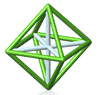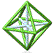Park City Mathematics Institute Functions Project Abstracts Drafts of Project Files (password required)

Parabola Investigations

The following four investigations showcase various methods to generate and explore parabolas.

Natural Parabolas
Lynda Waters

The purpose of this lesson is to use digital photography of "natural" parabolas to model quadratic functions. Once the digital pictures are downloaded to a computer, they can be sized to fit, exported, and imported onto Geometer's Sketchpad. By placing a coordinate grid onto the picture, the lesson continues with instructions on how to determine the equation of the function that fits the pictured parabola. Pictures of "natural" parabolas will give meaning to the mathematics.

Using Geometer's Sketchpad To Construct Parabolas With Focus & Directrix
Kelley Butler

By definition a parabola is the set of points in a plane that are the same distance from a fixed point - the focus - as from a fixed line - the directrix. In an effort to visually demonstrate the dynamic sketch, step-by-step instructions will be provided using Geometer's Sketchpad. Questions will also be included that are intended to clarify the relationships between the focus, directrix, and graph of any parabola.

An Introduction to Bézier Curves: Generating a Parabola From Three Points
Seth Bundy

Bézier curves are used in computer-aided geometric design. They were initially invented for car design but are commonly used today in font design. The general idea is that curves can be created when we use linear interpolation on line segments defined parametrically. To introduce these ideas to precalculus students, we explore how parabolic curves are created from three points connected by two line segments. We begin by doing string art and showing that the resulting curve is indeed a parabola. We proceed to a review of parametric equations, a foundational concept which will ground our work and provide an algebraic connection. An investigation on Geometer's Sketchpad illustrates how the parametric definition plays out graphically. Finally, some unproven conjectures are posed which will challenge students to utilize prior knowledge in making some discoveries and connections. These explorations will help to enrich this introduction for future use and to smooth the path toward more complicated Bézier curves.

Lab: Using Digital Video to Model Parabolas Parametrically
Connie Savoie

The purpose of this laboratory is to generate data and find the parametric equations to model each situation. The goal is to increase the students' working knowledge of parametric equations. Modeling them in real life will help students visualize what parametric equations really mean.

Students will generate lines and parabolas by rolling a ball across the graph paper while filming with a digital camera. Viewing the path of the ball while using the frame by frame progression allows points to be gathered with t, x and y. Parametric equations can be developed with these points, either using regression on the TI-83/84 or by hand. Further exploration can be made in several directions:

1. explore the effect of different slopes on the coefficient of the squared term
2. (in a calculus class) taking the first and second derivatives to explore velocity and acceleration
3. use trigonometry to find the angles of the slopes

Composing the Plane: Isometries as Graphs and Matrices
Rani Fischer

This unit examines the relationship between the graphs and matrices of isometries and compositions of isometries. Isometries are functions which transform objects in a way that changes neither size nor shape. Students are introduced to isometries: reflections, rotations, translations, and glide reflections with patty paper folding and tracing. From this experience they can construct these images on Geometer's Sketchpad and see a slightly different representation. Finally, students use trial and error first to formulate matrices for reflections over axes and rotations. Translations are shown both by vectors and by writing as 3x3 matrices. Other concepts that arise are line symmetry, symmetry groups, matrix multiplication with and without a calculator, and non-commutativity of matrix multiplication. This unit takes at least two weeks and assumes some familiarity with Geometer's Sketchpad software.

Petunia Has a Plan
Donna Curran, Marta Trimble, Celeste Williams
The working group project is centered around the subject of parabolas. The focus of this particular group is to create a multilevel approach to introducing parabolas which ranges from grade 8 to grade 10. It begins with a story that moves students through the process of forming generalizations and predictions based upon patterns drawn from the information to constructing tables and graphically depicting the information from those tables. In this process, the understanding of parabolas precipitates and is facilitated by questioning from and to the students.

Back to Functions IndexPCMI@MathForum Home || IAS/PCMI Home© 2001 - 2018 Park City Mathematics Institute IAS/Park City Mathematics Institute is an outreach program of the Institute for Advanced Study, 1 Einstein Drive, Princeton, NJ 08540 Send questions or comments to: Suzanne Alejandre and Jim King

With program support provided by Math for America

This material is based upon work supported by the National Science Foundation under DMS-0940733 and DMS-1441467. Any opinions, findings, and conclusions or recommendations expressed in this material are those of the author(s) and do not necessarily reflect the views of the National Science Foundation.